Theory and Modern Applications

# Periodic solutions of a quasilinear parabolic equation with nonlinear convection terms

## Abstract

In this paper, we study a periodic quasilinear parabolic equation with nonlinear convection terms and weakly nonlinear sources. Based on the theory of the Leray-Schauder fixed point theorem, we establish the existence of periodic solutions when the domain of the solution is sufficiently small.

## 1 Introduction

In this paper, we consider the following periodic quasilinear parabolic equation with nonlinear convection terms and weakly nonlinear sources: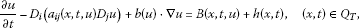(1.1)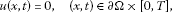(1.2)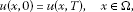(1.3)

where Ω is a bounded domain in ${\mathbb{R}}^{N}$ with a smooth boundary Ω, ${Q}_{T}=\mathrm{\Omega }×\left(0,T\right)$, and we assume that

(A1) ${a}_{ij}\left(\cdot ,\cdot ,u\right)={a}_{ji}\left(\cdot ,\cdot ,u\right)\in {C}_{T}\left({\overline{Q}}_{T}\right)$ and there exist two constants $0<\lambda \le \mathrm{\Lambda }$ such that

$\lambda {|\xi |}^{2}\le {a}_{ij}\left(x,t,u\right){\xi }_{i}{\xi }_{j}\le \mathrm{\Lambda }{|\xi |}^{2},\phantom{\rule{1em}{0ex}}\mathrm{\forall }\left(x,t\right)\in {Q}_{T},\xi \in {\mathbb{R}}^{+}.$

(A2) $B\left(x,t,u\right)$ is Hölder continuous in $\overline{\mathrm{\Omega }}×\mathbb{R}×\mathbb{R}$, periodic in t with a period T and satisfies $B\left(x,t,u\right)u\le {b}_{0}{|u|}^{\alpha +1}$ with constants ${b}_{0}\ge 0$ and $0\le \alpha \le 1$.

(A3) $h\left(x,t\right)\in {C}_{T}\left({\overline{Q}}_{T}\right)\cap {L}^{\mathrm{\infty }}\left(0,T;{W}_{0}^{1,\mathrm{\infty }}\left(\mathrm{\Omega }\right)\right)$, $h\left(x,t\right)>0$ for $\mathrm{\Omega }×\mathbb{R}$, where ${C}_{T}\left({\overline{Q}}_{T}\right)$ denotes the set of functions which are continuous in $\overline{\mathrm{\Omega }}×\mathbb{R}$ and ω-periodic with respect to t.

The existence of periodic solutions for parabolic equations has been considered by several authors; see  and the references therein. As a work related to this paper, we refer to Nakao , in which the author considered the following parabolic equation:

$\frac{\partial u}{\partial t}-\mathrm{\Delta }\beta \left(u\right)=B\left(x,t,u\right)+h\left(x,t\right),$

with Dirichlet boundary value conditions, where B, h are periodic in t with a period $\omega >0$, $\beta \left(u\right)$ satisfies ${\beta }^{\prime }\left(u\right)>0$ except for $u=0$ and $\beta \left(u\right)$ is fulfilled by ${|u|}^{m-1}u$ if $m>1$. Under the assumption that $B\left(x,t,u\right)u\le {b}_{0}|u|$, Nakao established the existence of periodic solutions by the Leray-Schauder fixed point theorem. In , Zhou et al. considered the quasilinear parabolic equation with nonlocal terms. Based on the theory of Leray-Schauder’s degree, the authors established the existence of nontrivial periodic solutions. In this paper, we consider the quasilinear parabolic equation (1.1) with weakly nonlinear sources and nonlinear convection terms. The convection term $b\left(u\right)\cdot \mathrm{\nabla }u$ describes an effect of convection with a velocity field $b\left(u\right)$. Under a restrictive condition that the domain is sufficiently small, we establish the existence of periodic solutions of the problem (1.1)-(1.3).

This paper is organized as follows. The definition of the generalized solution and a useful a priori estimate are presented in Section 2. Our main results will be given in Section 3.

## 2 Preliminaries

Our main efforts will focus on the discussion of generalized solutions since the regularity follows from a quite standard approach. Hence, we give the following definition of generalized solutions.

Definition 1 A function u is said to be a generalized solution of the problem (1.1)-(1.3) if $u\in {L}^{2}\left(0,T;{H}_{0}^{1}\left(\mathrm{\Omega }\right)\right)\cap {C}_{T}\left({\overline{Q}}_{T}\right)$ and

${\iint }_{{Q}_{T}}\left(-u\frac{\partial \phi }{\partial t}+{a}_{ij}\left(x,t,u\right){D}_{i}u{D}_{j}\phi -\beta \left(u\right)\cdot \mathrm{\nabla }\phi -B\left(x,t,u\right)\phi -h\left(x,t\right)\phi \right)\phantom{\rule{0.2em}{0ex}}dx\phantom{\rule{0.2em}{0ex}}dt=0$
(2.1)

for any $\phi \in {C}^{1}\left({\overline{Q}}_{T}\right)$ with $\phi \left(x,0\right)=\phi \left(x,T\right)$ and $\phi {|}_{\partial \mathrm{\Omega }×\left(0,T\right)}=0$, where $\beta \left(u\right)=\left({\beta }_{1}\left(u\right),\dots ,{\beta }_{N}\left(u\right)\right)$ and ${\beta }_{i}\left(u\right)={\int }_{0}^{u}{b}_{i}\left(s\right)\phantom{\rule{0.2em}{0ex}}ds$, $i=1,\dots ,N$.

For convenience, we let ${\parallel \cdot \parallel }_{p}$ and ${\parallel \cdot \parallel }_{m,p}$ denote ${L}^{p}\left(\mathrm{\Omega }\right)$ and ${W}^{m,p}\left(\mathrm{\Omega }\right)$ norms, respectively. First, we establish the following a priori estimate which plays an important role in the proof of the main results of this paper.

Lemma 1 Let u be a solution of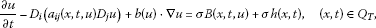(2.2)(2.3)(2.4)

with $\sigma \in \left[0,1\right]$, then there exists a positive constant R independent of σ such that

${\parallel u\left(t\right)\parallel }_{{L}^{\mathrm{\infty }}\left({Q}_{T}\right)}
(2.5)

when the measure of Ω is small enough.

Proof Suppose u is a solution of the problem (2.2)-(2.4). Multiplying equation (2.2) by ${|u|}^{p}u$ ($p\ge 0$) and integrating the resulting relation over Ω, noticing that

$\begin{array}{rcl}{\int }_{\mathrm{\Omega }}b\left(u\right)\cdot \mathrm{\nabla }u{|u|}^{p}u\phantom{\rule{0.2em}{0ex}}dx& =& {\int }_{\mathrm{\Omega }}\sum _{i=1}^{N}{b}_{i}\left(u\right){|u|}^{p}u\frac{\partial u}{\partial {x}_{i}}\phantom{\rule{0.2em}{0ex}}dx=\sum _{i=1}^{N}{\int }_{\mathrm{\Omega }}{\left({\int }_{0}^{u}{b}_{i}\left(s\right){|s|}^{p}s\phantom{\rule{0.2em}{0ex}}ds\right)}_{{x}_{i}}\phantom{\rule{0.2em}{0ex}}dx\\ =& \sum _{i=1}^{N}{\int }_{\partial \mathrm{\Omega }}\left({\int }_{0}^{u}{b}_{i}\left(s\right){|s|}^{p}s\phantom{\rule{0.2em}{0ex}}ds\right)cos\left(\mathrm{n},{x}_{i}\right)\phantom{\rule{0.2em}{0ex}}dx=0,\end{array}$

where n is the outer normal to Ω, we have(2.6)

The second term of the left-hand side in the above integral equality can be written as

$\begin{array}{rcl}-{\int }_{\mathrm{\Omega }}{D}_{i}\left({a}_{ij}\left(x,t,u\right){D}_{j}u\right){|u\left(t\right)|}^{p}u\left(t\right)\phantom{\rule{0.2em}{0ex}}dx& =& \left(p+1\right){\int }_{\mathrm{\Omega }}{|u\left(t\right)|}^{p}{a}_{ij}\left(x,t,u\right){D}_{j}u{D}_{i}u\phantom{\rule{0.2em}{0ex}}dx\\ \ge & \frac{4\lambda \left(p+1\right)}{{\left(p+2\right)}^{2}}{\int }_{\mathrm{\Omega }}|\mathrm{\nabla }\left[{|u\left(t\right)|}^{\frac{p}{2}}u\left(t\right)\right]{|}^{2}\phantom{\rule{0.2em}{0ex}}dx,\end{array}$

and

${\int }_{\mathrm{\Omega }}{|u\left(t\right)|}^{p}u\left(t\right)h\phantom{\rule{0.2em}{0ex}}dx\le {\left({\int }_{\mathrm{\Omega }}{|u\left(t\right)|}^{p+2}\phantom{\rule{0.2em}{0ex}}dx\right)}^{\frac{p+1}{p+2}}{\left({\int }_{\mathrm{\Omega }}{h}^{p+2}\phantom{\rule{0.2em}{0ex}}dx\right)}^{\frac{1}{p+2}}.$

Hence, from (2.6), we have

$\frac{d}{dt}{\parallel u\left(t\right)\parallel }_{p+2}^{p+2}+{C}_{1}{\parallel \mathrm{\nabla }\left({|u\left(t\right)|}^{\frac{p}{2}}u\left(t\right)\right)\parallel }_{2}^{2}\le {C}_{2}\left(p+2\right)\left({\parallel u\left(t\right)\parallel }_{p+\alpha +1}^{p+\alpha +1}+{\parallel u\parallel }_{p+2}^{p+1}\right),$
(2.7)

where ${C}_{1}$, ${C}_{2}$ are positive constants independent of $u\left(t\right)$, p.

If $0\le \alpha <1$, by Hölder’s inequality and Young’s inequality, we have

$\begin{array}{rcl}{\int }_{\mathrm{\Omega }}{|u\left(t\right)|}^{p+\alpha +1}\phantom{\rule{0.2em}{0ex}}dx& \le & {\left({\int }_{\mathrm{\Omega }}{|u\left(t\right)|}^{p+2}\phantom{\rule{0.2em}{0ex}}dx\right)}^{\frac{p+\alpha +1}{p+2}}{|\mathrm{\Omega }|}^{\frac{1-\alpha }{p+2}}\\ \le & max\left\{1,{|\mathrm{\Omega }|}^{\frac{1}{2}}\right\}{\parallel u\left(t\right)\parallel }_{p+2}^{p+\alpha +1}\\ =& max\left\{1,{|\mathrm{\Omega }|}^{\frac{1}{2}}\right\}{\parallel u\left(t\right)\parallel }_{p+2}^{\left(p+2\right)\alpha }{\parallel u\left(t\right)\parallel }_{p+2}^{\left(p+1\right)\left(1-\alpha \right)}\\ \le & {\parallel u\left(t\right)\parallel }_{p+2}^{p+2}+C{\parallel u\left(t\right)\parallel }_{p+2}^{p+1}.\end{array}$
(2.8)

Combined with (2.7), it yields

$\frac{d}{dt}{\parallel u\left(t\right)\parallel }_{p+2}^{p+2}+{C}_{1}{\parallel \mathrm{\nabla }\left({|u\left(t\right)|}^{\frac{p}{2}}u\left(t\right)\right)\parallel }_{2}^{2}\le {C}_{2}\left(p+2\right)\left({\parallel u\left(t\right)\parallel }_{p+2}^{p+2}+{\parallel u\left(t\right)\parallel }_{p+2}^{p+1}\right).$
(2.9)

If $\alpha =1$, from (2.7) we can get (2.9) directly.

Set

${u}_{k}\left(t\right)={|u\left(t\right)|}^{\frac{{p}_{k}}{2}}u\left(t\right),\phantom{\rule{1em}{0ex}}{p}_{k}={2}^{k}-2\phantom{\rule{0.25em}{0ex}}\left(k=1,2,\dots \right),$

then ${p}_{k}=2{p}_{k-1}+2$. From (2.9), we have

$\frac{d}{dt}{\parallel {u}_{k}\left(t\right)\parallel }_{2}^{2}+{C}_{1}{\parallel \mathrm{\nabla }{u}_{k}\left(t\right)\parallel }_{2}^{2}\le {C}_{2}\left({p}_{k}+2\right){\parallel {u}_{k}\left(t\right)\parallel }_{2}^{2}+{C}_{2}\left({p}_{k}+2\right){\parallel {u}_{k}\left(t\right)\parallel }_{2}^{\frac{2\left({p}_{k}+1\right)}{{p}_{k}+2}}.$

By the Gagliardo-Nirenberg inequality, we have

(2.10)

Noticing ${\parallel {u}_{k}\left(t\right)\parallel }_{1}={\parallel {u}_{k-1}\left(t\right)\parallel }_{2}^{2}$, by (2.10) we obtain

$\begin{array}{rcl}\frac{d}{dt}{\parallel {u}_{k}\left(t\right)\parallel }_{2}^{2}& \le & -{C}_{1}{\parallel {u}_{k}\left(t\right)\parallel }_{2}^{\frac{2}{\theta }}{\parallel {u}_{k}\left(t\right)\parallel }_{1}^{\frac{2\left(\theta -1\right)}{\theta }}+{C}_{2}\left({p}_{k}+2\right){\parallel {u}_{k}\left(t\right)\parallel }_{2}^{2}\\ +{C}_{2}\left({p}_{k}+2\right){\parallel {u}_{k}\left(t\right)\parallel }_{2}^{\frac{2\left({p}_{k}+1\right)}{{p}_{k}+2}}\\ \le & -{C}_{1}{\parallel {u}_{k}\left(t\right)\parallel }_{2}^{\frac{2}{\theta }}{\parallel {u}_{k-1}\left(t\right)\parallel }_{2}^{\frac{4\left(\theta -1\right)}{\theta }}+{C}_{2}\left({p}_{k}+2\right){\parallel {u}_{k}\left(t\right)\parallel }_{2}^{2}\\ +{C}_{2}\left({p}_{k}+2\right){\parallel {u}_{k}\left(t\right)\parallel }_{2}^{\frac{2\left({p}_{k}+1\right)}{{p}_{k}+2}}.\end{array}$
(2.11)

Set ${\lambda }_{k}=max\left\{1,{sup}_{t}{\parallel {u}_{k}\left(t\right)\parallel }_{2}\right\}$, then

$\begin{array}{rcl}\frac{d}{dt}{\parallel {u}_{k}\left(t\right)\parallel }_{2}^{2}& \le & {\parallel {u}_{k}\left(t\right)\parallel }_{2}^{\frac{2\left({p}_{k}+1\right)}{{p}_{k}+2}}\left\{-{C}_{1}{\parallel {u}_{k}\left(t\right)\parallel }_{2}^{\frac{2}{\theta }-\frac{2\left({p}_{k}+1\right)}{{p}_{k}+2}}{\lambda }_{k-1}^{\frac{4\left(\theta -1\right)}{\theta }}\\ +{C}_{2}\left({p}_{k}+2\right){\parallel {u}_{k}\left(t\right)\parallel }_{2}^{\frac{2}{{p}_{k}+2}}+{C}_{2}\left({p}_{k}+2\right)\right\}.\end{array}$
(2.12)

Now, we estimate $\left({p}_{k}+2\right){\parallel {u}_{k}\left(t\right)\parallel }_{2}^{\frac{2}{{p}_{k}+2}}$. By Young’s inequality,

$ab\le ϵ{a}^{{p}^{\prime }}+{ϵ}^{-\frac{{q}^{\prime }}{{p}^{\prime }}}\frac{1}{{q}^{\prime }}{\left(\frac{1}{{p}^{\prime }}\right)}^{\frac{{q}^{\prime }}{{p}^{\prime }}}{b}^{{q}^{\prime }},$

where ${p}^{\prime }>1$, ${q}^{\prime }>1$, $\frac{1}{{p}^{\prime }}+\frac{1}{{q}^{\prime }}=1$ with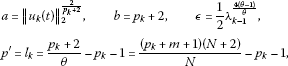we have

$\left({p}_{k}+2\right){\parallel {u}_{k}\left(t\right)\parallel }_{2}^{\frac{2}{{p}_{k}+2}}\le \frac{1}{2}{\parallel u\parallel }_{2}^{\frac{2}{\theta }-\frac{2\left({p}_{k}+1\right)}{{p}_{k}+2}}{\lambda }_{k-1}^{\frac{4\left(\theta -1\right)}{\theta }}+C{\left({p}_{k}+2\right)}^{\frac{{l}_{k}}{{l}_{k}-1}}{\lambda }_{k-1}^{\frac{4\left(1-\theta \right)}{\theta \left({l}_{k}-1\right)}}.$
(2.13)

It is easy to see that ${lim}_{k\to \mathrm{\infty }}{l}_{k}=+\mathrm{\infty }$. Denote

${a}_{k}=\frac{{l}_{k}}{{l}_{k}-1},\phantom{\rule{2em}{0ex}}{b}_{k}=\frac{4\left(1-\theta \right)}{\theta \left({l}_{k}-1\right)}.$

From (2.12), (2.13), we have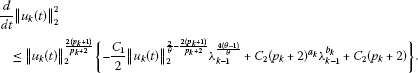That is,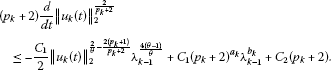(2.14)

The periodicity of ${u}_{k}\left(t\right)$ implies that there exists ${t}^{\prime }$ such that ${\parallel {u}_{k}\left(t\right)\parallel }_{2}$ takes its maximum and the left-hand side of (2.14) vanishes. Then we have

${\parallel {u}_{k}\left(t\right)\parallel }_{2}\le {\left\{C\left[\left({p}_{k}+2\right)+{\left({p}_{k}+2\right)}^{{a}_{k}}{l}_{k-1}^{{b}_{k}}\right]{\lambda }_{k-1}^{\frac{4\left(1-\theta \right)}{\theta }}\right\}}^{\frac{1}{{c}_{k}}},$

where

${c}_{k}=\frac{2}{\theta }-\frac{2\left({p}_{k}+1\right)}{{p}_{k}+2}=\frac{2{l}_{k}}{{p}_{k}+2}.$

Since ${\lambda }_{k-1}\ge 1$ ($k=1,2,\cdot$), it follows that

${\parallel {u}_{k}\left(t\right)\parallel }_{2}\le {\left\{C{\left({p}_{k}+2\right)}^{{a}_{k}}{\lambda }_{k-1}^{{b}_{k}+\frac{4\left(1-\theta \right)}{\theta }}\right\}}^{\frac{1}{{c}_{k}}}={\left\{C{\left({p}_{k}+2\right)}^{{a}_{k}}\right\}}^{\frac{{p}_{k}+2}{2{l}_{k}}}{\lambda }_{k-1}^{\frac{4\left(1-\theta \right)\left({p}_{k}+2\right)}{2\left({l}_{k}-1\right)\theta }}.$

Noticing that $\frac{{p}_{k}+2}{\left({l}_{k}-1\right)\theta }=\frac{1}{1-\theta }$ and $\frac{{p}_{k}+2}{2{l}_{k}}$ are bounded, we have

${\parallel {u}_{k}\left(t\right)\parallel }_{2}\le C{2}^{k{a}^{\prime }}{\lambda }_{k-1}^{2},$

where ${a}^{\prime }$ is a positive constant independent of k. That is,

$ln{\parallel {u}_{k}\left(t\right)\parallel }_{2}\le ln{l}_{k}\le lnC+klnA+2ln{l}_{k-1},$

where $A={2}^{{a}^{\prime }}>1$, then

$\begin{array}{rcl}ln{\parallel {u}_{k}\left(t\right)\parallel }_{2}& \le & lnC\sum _{i=0}^{k-2}{2}^{i}+{2}^{k-1}ln{\lambda }_{1}+lnA\left(\sum _{j=0}^{k-2}\left(k-j\right){2}^{j}\right)\\ \le & \left({2}^{k-1}-1\right)lnC+{2}^{k-1}ln{\lambda }_{1}+f\left(k\right)lnA\end{array}$

with

$f\left(k\right)={2}^{k+1}-{2}^{k-1}-k-2.$

That is,

${\parallel u\left(t\right)\parallel }_{{p}_{k}+2}\le {\left\{{C}^{{2}^{k-1}}{\lambda }_{1}^{{2}^{k-1}}{A}^{f\left(k\right)}\right\}}^{\frac{2}{{p}_{k}+2}}.$

Letting $k\to \mathrm{\infty }$, we obtain

${\parallel u\left(t\right)\parallel }_{\mathrm{\infty }}\le C{\lambda }_{1}^{2}\le C{\left(max\left\{1,\underset{t}{sup}{\parallel u\left(t\right)\parallel }_{2}\right\}\right)}^{2}.$
(2.15)

In order to estimate ${\parallel u\left(t\right)\parallel }_{2}$, we set $p=0$. From (2.9), we obtain

$\frac{d}{dt}{\parallel u\left(t\right)\parallel }_{2}^{2}+{C}_{1}{\parallel \mathrm{\nabla }u\left(t\right)\parallel }_{2}^{2}\le {C}_{2}{\parallel u\left(t\right)\parallel }_{2}^{2}+{C}_{2}{\parallel u\left(t\right)\parallel }_{2}.$

According to the Poincaré inequality, we have

${C}_{p}{\parallel u\left(t\right)\parallel }_{2}^{2}\le {\parallel \mathrm{\nabla }u\left(t\right)\parallel }_{2}^{2},$

where ${C}_{p}$ is a positive constant which depends only on N and the measure of Ω and becomes very large when $|\mathrm{\Omega }|$ becomes small. Then

$\frac{d}{dt}{\parallel u\left(t\right)\parallel }_{2}^{2}+{C}_{1}{C}_{p}{\parallel u\left(t\right)\parallel }_{2}^{2}\le {C}_{2}{\parallel u\left(t\right)\parallel }_{2}^{2}+{C}_{2}{\parallel u\left(t\right)\parallel }_{2}.$

So, when $|\mathrm{\Omega }|$ is sufficiently small, we have ${C}_{1}{C}_{p}>{C}_{2}$. Then by Young’s inequality, we obtain

$\frac{d}{dt}{\parallel u\left(t\right)\parallel }_{2}^{2}+C{\parallel u\left(t\right)\parallel }_{2}^{2}\le C,$

where C is a constant independent of u. By the periodicity of u, we have

${\parallel u\left(t\right)\parallel }_{2}\le R,$

where R is a positive constant independent of σ. Combining the above inequality with (2.15), we obtain (2.5). The proof is completed. □

## 3 The main results

Our main result is the following theorem.

Theorem 1 If (A1), (A2) and (A3) hold, then the problem (1.1)-(1.3) admits at least one periodic solution u.

Proof First, we define a map by considering the following problem: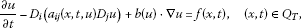(3.1)(3.2)(3.3)

where $f\left(x,t\right)$ is a given function in ${C}_{T}\left({\overline{Q}}_{T}\right)$. It follows from a standard argument similar to  that the problem (3.1)-(3.3) admits a unique solution. So, we can define a map $T:{C}_{T}\left({\overline{Q}}_{T}\right)\to {C}_{T}\left({\overline{Q}}_{T}\right)$ by $u=Tf$ and the map $u=Tf$ is compact and continuous. In fact, by the method in , we can infer that ${\parallel u\parallel }_{{L}^{\mathrm{\infty }}\left({Q}_{T}\right)}$ is bounded if $f\in {L}^{\mathrm{\infty }}\left({Q}_{T}\right)$ and u, $\mathrm{\nabla }u\in {C}^{\alpha }\left(\overline{{Q}_{T}}\right)$ for some $\alpha >0$. Then (by the Arzela-Ascoli theorem) the compactness of the map T comes from ${\parallel u\parallel }_{{L}^{\mathrm{\infty }}\left({Q}_{T}\right)}$ and the Hölder continuity of u. The continuity of the map T comes from the Hölder continuity of u.

Let $\mathrm{\Phi }\left(u\right)=B\left(x,t,u\right)+h\left(x,t\right)$, by (A2)-(A3) and the above arguments, we see that $T\left(\sigma \mathrm{\Phi }\right)$ is a complete continuous map for $\sigma \in \left[0,1\right]$. By Lemma 1, we can see that any fixed point u of the map $T\left(\sigma \mathrm{\Phi }\right)$ satisfies

${\parallel u\parallel }_{\mathrm{\infty }}\le C,$

where C is a positive constant independent of σ. Then, by the Leray-Schauder fixed point theorem , we conclude that the problem (1.1)-(1.3) admits a periodic solution u. The proof is complete. □

## References

1. Alaa N, Iguernane M: Weak periodic solutions of some quasilinear parabolic equations with data measures. J. Inequal. Pure Appl. Math. 2002., 3(3): Article ID 46

2. Badii M: Periodic solutions for a class of degenerate evolution problems. Nonlinear Anal. 2001, 44(4):499–508. 10.1016/S0362-546X(99)00277-1

3. Badii M, Diaz JI: Periodic solutions of a quasilinear parabolic boundary value problem arising in unsaturated flow through a porous medium. Appl. Anal. 1995, 56: 279–301. 10.1080/00036819508840327

4. Dibenedette E, Friedman A: Periodic behaviour for the evolutionary dam problem and related free boundary problems evolutionary dam problem. Commun. Partial Differ. Equ. 1986, 11(12):1297–1377. 10.1080/03605308608820466

5. Khoma LG, Khoma NG: Generalized periodic solutions of quasilinear equations. Ukr. Math. J. 1996, 48(3):453–459. 10.1007/BF02378534

6. Lieberman GM: Time-periodic solutions of quasilinear parabolic differential equations: I. Dirichlet boundary conditions. J. Math. Anal. Appl. 2001, 264(2):617–638. 10.1006/jmaa.2000.7145

7. Liu ZH: Periodic solutions for double degenerate quasilinear parabolic equations. Nonlinear Anal. 2002, 51(7):1245–1257. 10.1016/S0362-546X(01)00893-8

8. Liu BP, Pao CV: Periodic solutions of coupled semilinear parabolic boundary value problems. Nonlinear Anal. 1982, 6(3):237–252. 10.1016/0362-546X(82)90092-X

9. Ladyzenskaja OA, Solonnikov VA, Ural’ceva NN Translation of Mathematical Monographs 23. In Linear and Quasilinear Equations of Parabolic Type. Am. Math. Soc., Providence; 1968.

10. Smulev II: Periodic solutions of the first boundary value problem for parabolic equations. Am. Math. Soc. Transl. Set 1969, 79(2):215–229.

11. Zhang QY, Lin ZG: Periodic solutions of quasilinear parabolic systems with nonlinear boundary conditions. Nonlinear Anal. TMA 2010, 72: 3429–3435. 10.1016/j.na.2009.12.026

12. Zhou Q, Huang R, Ke YY, Wang YF: Existence of the nontrivial nonnegative periodic solutions for the quasilinear parabolic equation with nonlocal terms. Comput. Math. Appl. 2005, 50: 1293–1302. 10.1016/j.camwa.2005.06.005

13. Guo DG: Nonlinear Functional Analysis. Shandong Science and Technology Press, Jinan; 2001.

## Acknowledgements

The authors are grateful to the associate editor and the referees for their helpful suggestions to improve some results in this paper. This work is supported by the National Natural Science Foundation of China (71173060, 70773028, 71031003) and the Fundamental Research for the Central Universities (Grant No. HIT. HSS. 201201).

## Author information

Authors

### Corresponding author

Correspondence to Songsong Li.

### Competing interests

The authors declare that they have no competing interests.

### Authors’ contributions

SL and XH carried out the proof of the main part of this article, XH corrected the manuscript and participated in its design and coordination. All authors have read and approved the final manuscript.

## Rights and permissions

Reprints and Permissions

Li, S., Hui, X. Periodic solutions of a quasilinear parabolic equation with nonlinear convection terms. Adv Differ Equ 2012, 206 (2012). https://doi.org/10.1186/1687-1847-2012-206

• Accepted:

• Published:

• DOI: https://doi.org/10.1186/1687-1847-2012-206

### Keywords

• Positive Constant
• Generalize Solution
• Periodic Solution
• Parabolic Equation
• Dirichlet Boundary